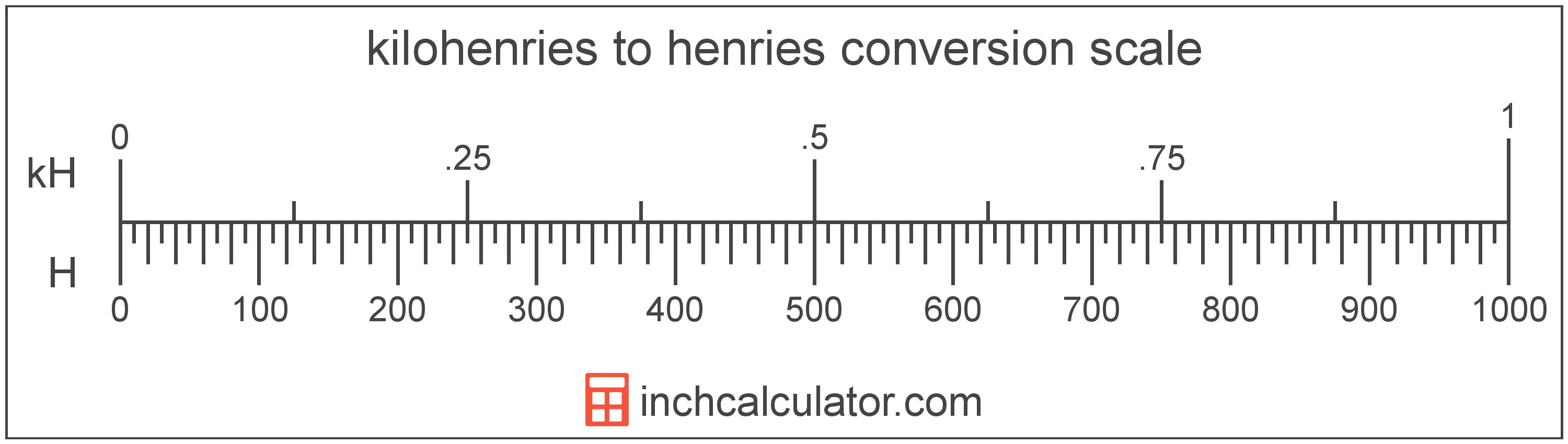# Henries to Kilohenries Converter

Enter the electrical inductance in henries below to get the value converted to kilohenries.

Results in Kilohenries:1 H = 0.001 kH

Do you want to convert kilohenries to henries?

## How to Convert Henries to Kilohenries

To convert a measurement in henries to a measurement in kilohenries, divide the electrical inductance by the following conversion ratio: 1,000 henries/kilohenry.

Since one kilohenry is equal to 1,000 henries, you can use this simple formula to convert:

kilohenries = henries ÷ 1,000

The electrical inductance in kilohenries is equal to the electrical inductance in henries divided by 1,000.

For example, here's how to convert 5,000 henries to kilohenries using the formula above.
kilohenries = (5,000 H ÷ 1,000) = 5 kH## What is a Henry?

One henry is equal to the inductance of a conductor in which there is one volt of electromotive force when the current through the conductor is increased by one ampere per second.

The henry is the SI derived unit for electrical inductance in the metric system. Henries can be abbreviated as H; for example, 1 henry can be written as 1 H.

The National Institute of Standards and Technology recommends using "henries" as the plural form of the unit, however the usage of "henrys" is also common.

## What is a Kilohenry?

One kilohenry is equal to 1,000 henries, which are the inductance of a conductor with one volt of electromotive force when the current is increased by one ampere per second.

The kilohenry is a multiple of the henry, which is the SI derived unit for electrical inductance. In the metric system, "kilo" is the prefix for thousands, or 103. Kilohenries can be abbreviated as kH; for example, 1 kilohenry can be written as 1 kH.

## Henry to Kilohenry Conversion Table

Table showing various henry measurements converted to kilohenries.
Henries Kilohenries
1 H 0.001 kH
2 H 0.002 kH
3 H 0.003 kH
4 H 0.004 kH
5 H 0.005 kH
6 H 0.006 kH
7 H 0.007 kH
8 H 0.008 kH
9 H 0.009 kH
10 H 0.01 kH
20 H 0.02 kH
30 H 0.03 kH
40 H 0.04 kH
50 H 0.05 kH
60 H 0.06 kH
70 H 0.07 kH
80 H 0.08 kH
90 H 0.09 kH
100 H 0.1 kH
200 H 0.2 kH
300 H 0.3 kH
400 H 0.4 kH
500 H 0.5 kH
600 H 0.6 kH
700 H 0.7 kH
800 H 0.8 kH
900 H 0.9 kH
1,000 H 1 kH

## References

1. International Bureau of Weights and Measures, The International System of Units, 9th Edition, 2019, https://www.bipm.org/documents/20126/41483022/SI-Brochure-9-EN.pdf
2. Ambler Thompson and Barry N. Taylor, Guide for the Use of the International System of Units (SI), National Institute of Standards and Technology, https://physics.nist.gov/cuu/pdf/sp811.pdf